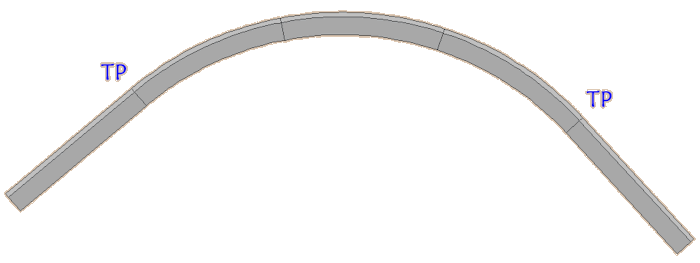On This Page...Related Pages

## Introduction

This page shows how the size of the radius of a kerb, whether it's an individual kerbstone or an extant arc, can be determined using a simple bit of mathematics.
Every now and again, it becomes necessary to determine the radius of a kerb or an arced kerb line. It may be that the kerb is unmarked or the marking has weathered away and a radius kerb of a particular size is required. Or it may be that an existing arc of kerbing neds to be replaced, and the actual size of the radius isn't known.## Quarter Circle arcs:

When an existing line of kerbs forms a quarter-circle (which is a 90° arc), then determining the radius is incredibly simple: the distance from tangent point to the interception of a straight line projected from the other tangent point *must* be the same as the radius of the arc.If the straight kerb lines, marked as A-TP1 and B-TP2 on the sketch above, are projected (by pulling string lines along them, for example), the distance between the Tangent Point (TP) to the intersection of the projected lines *must* be the same as the distance between the origin of the arc and the face of the kerb, that is, the radius of the arc.## Mathematical determination:

There are many more instances where the angle covered by the arc is NOT a highly convenient quarter -circle (90°) but is more acute (less than 90°) or more obtuse (greater than 90deg;). Luckily, there is a constant and simple mathematical relationship between the length of a chord strung between two points on the arc, and the distance of the centre point of that chord to the intersection point on the arc of a perpendicular line from that mid-point. It's not as complicated as it sounds!

Two points to note, though, before beginning with this technique:

• 1 - The maths behind this technique works on any true arc whether it's an individual kerb or an extended arc.
• 2 - It's best to subtend the chord from two points on the arc that are located at points separated by an angle of less than 180°.

Here's the basic premise.....To find radius (r) of arc...

Create a chord, here labelled C - D, and measure its length.
Divide by 2 and refer to as "A"

Measure perpendicular distance from arc to mid-point of chord. Refer to as "B"

Add together the square of A plus the square of B

Divide the result by 2B (twice what was measured as a perpendicular)

Here's a worked example.....

An existing kerb arc has been badly damaged by road traffic and needs to be replaced. The layout is shown below.

The radius of the kerb arc needs to be established so that the correct size (radius) of replacement kerbs can be ordered from the supplier.The arc of kerbs isn't too large, and so it's decided to create the chord (referred to in geometry as "subtending" a chord) from tangent point to tangent point (TP1 to TP2).

Kerbs are manufactured to create fairly accurate arcs where the declared radius is the distance from the Origin of the arc to the face of the kerb. Consequently, it's best to arrange all measurements accordingly, that is, to and from the face of the kerb.

Although the geometry should still work if measurements are made using the rear face (back) of a kerb, the fact that there can be significant difference in the width of kerbs (even those manufactured using high-quality concrete moulds) could result in inaccuracy in the final calculated result.There's much more about the geometry used when setting out kerbs and paving on the Paving Geometry page Back to the job in hand..... The chord is subtended from tangent point to tangent point as shown.........and is measured. The distance from TP1 to TP2 is recorded as 2520mm On larger arcs or kerbs (say above radius = 600mm), there's no real need for millimetre accuracy when measuring the length of the chord or the perpendicular. The nearest centimetre (10mm) will be more than adequate. The mid-point of the chord is marked. This should result in the distance TP1 to mid-point = distance from TP2 to mid-point = 1260mm, as shown below. The value of half the chord length (1260mm in this case) is recorded as value A.The next required value is B, the distance from the chord mid-point to the face of the kerb measured perpendicular (ie: at 90°) to the chord. While it might be possible to set up a right-angled (90°) line from the chord to the kerb face, a reasonable assessment is to measure the maximum distance from the chord mid-point to the kerb face and that *should* be the same as a perpendicular measurement.In this example, the length of the perpendicular is, roughly, 520mm, as shown on the sketch above. We now have value B and all the information needed to determine the size of the kerb arc radius.The equation used to determine the radius was given previously, but is shown again opposite....The values for A and B were measured above, so it's known that..... A = 1260mm ...and... B = 520mm Substituting these values into the equation gives......and this is expanded to........which leads to........which can be worked out to a simpler....So, the radius is calculated as being 1,786.5mm (all the calculations were done in millimetres, but could have been done in metres, if preferred), which is ridiculously precise but indicates that, in all probability, kerbs with a radius of 1.8m (1,800mm) were originally used. So, that's what can now be ordered from the supplier!### Related pages on this site